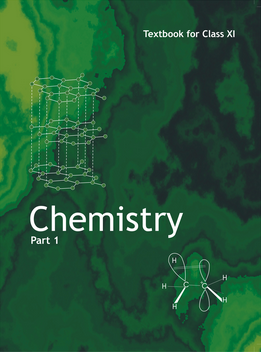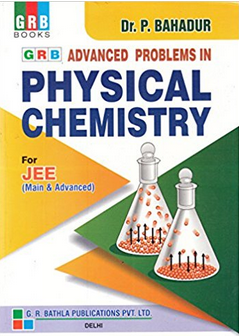# Some basic concepts in chemistry (Weightage 5%)   Share

### Topics from Some basic concepts in chemistry

• Precision and accuracy, significant figures, S.I. Units, dimensional analysis (9 concepts)
• Concept of atom, molecule, element and compund (5 concepts)
• Physical quantities and their measurements in chemistry (4 concepts)
• Percentage composition, Empirical and molecular formulae (2 concepts)
• Chemical equations and stiochiometry (7 concepts)
• Atomic and molecular masses, mole concept, molar mass (5 concepts)
• Matter and its nature (2 concepts)
• Dalton's atomic theory (4 concepts)
• Laws of chemical combination (4 concepts)
• Importance of chemistry and Nature of matter (2 concepts)
• Volume Strength of H2O2 Solution and Hardness of Water (1 concepts)
• n-Factor or Valence Factor (1 concepts)
• Uncertainty in measurement (1 concepts)
• Fundamental Laws of Chemical combinations (1 concepts)
• Percentage Composition (2 concepts)
• Dalton’s Atomic Model (1 concepts)
• Atomic And Molecular Masses (1 concepts)
• Mole Concept And Molar Masses (1 concepts)
• Stoichiometry, Stoichiometric Calculations (3 concepts)
• Smart Tips: Some Basic Concept in Chemistry (6 concepts)

### Important Books for Some basic concepts in chemistry

••Exams
Articles
Questions